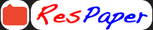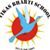Trending ▼   ResFinder# ISC Class XII Prelims 2019 : Computer Science (Vikas Bharti School, Gorakhpur)

8 pages, 9 questions, 0 questions with responses, 0 total responses,00Vikas Bharti +Fave Message ProfileTimelineUploads
 Home > sanjeevvbs >   F Also featured on: School PageFormatting page ...

VIKAS BHARTI SCHOOL BHARTIPURAM, GORAKHPUR Mock Trials 2019 (Set - A) COMPUTER SCIENCE (Form Sc) PAPER 1 (Theory) (Maximum marks: 70) (Time allowed: Three hours) (Candidates are allowed additional 15 minutes for only reading the paper, they must NOT start writing during this time) Answer all questions in part I(compulsory) and six questions from Part II, choosing two questions from Section A, two from Section B and two from Section C. All working, including rough work, should be done on the same sheet as the rest of the answer. The intended marks for questions or parts of questions are given in brackets [ ]. PART I (20 Marks) Answer ALL questions While answering questions in this part. Indicates briefly your working and reasoning, wherever required. Question: 1 a) Show that X ~ (Y X) is a Tautology.  b) Calculate the time complexity using Big O notation for the following given code segment:  for (int i=0;i<n;i++) s+=k; c) If F(A, B, C) = A (BC +B C), then find F .  d) Verify the following proposition with the help of a Truth Table.  P (~ P Q ) = P Q e) Convert the following infix expression into its postfix form  X + ( Y + Z ) + ( ( P + Q) * R ) / S Question: 2 a) The array D[m][n] is stored with each element requiring 4 bytes of storage. If the base address at D is 1500 and the address at D is 1608. Determine the number of rows of the matrix when the matrix is stored in Column Major Wise.  b) State how a binary tree is a recursive data structure?Formatting page ...Formatting page ...Formatting page ...Formatting page ...Formatting page ...Formatting page ...Formatting page ...